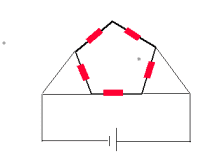# The total resistance in a circuit

• Aristarchus_

#### Aristarchus_

Homework Statement
What is the total resistance in this circuit, considering that each resistor has a resistance of $1$ Ohm
The correct answer is $2/3$ but I don't know how to obtain it

I thought one could combine the upper two resistors(in series) and combine them with the lower three such that they are in parallel. Then, $R=(1/2+1/3)^{-1}$

I just thought about something, If we split the resistor (by symmetry) we have, following the correct answer, three resistors on each side, which are parallel to each other. Thus $(1+1+1)^{-1} + (1+1+1)^{-1}$. There is just one problem with this, and it is the fact that the bottom resistor would by intuition be split in 1/2. Or is that not so?
Relevant Equations
R•Delta2

Homework Statement:: What is the total resistance in this circuit, considering that each resistor has a resistance of $1$ Ohm
Relevant Equations:: R

View attachment 305115
What is your work so far?
You are required to provide effort according to the homework guidelines.

I thought one could combine the upper three resistors(in series) and combine them with the lower three such that they are in parallel. Then, $R=(1/2+1/3)^{-1}$

I thought one could combine the upper three resistors(in series) and combine them with the lower three such that they are in parallel. Then, $R=(1/2+1/3)^{-1}$
Do you mean the upper two?

Then it would be correct if not for the lower wires connecting in between the lower three resistors. So what is the potential difference between the two connection points for each side?

Do you mean the upper two?

Then it would be correct if not for the lower wires connecting in between the lower three resistors. So what is the potential difference between the two connection points for each side?
Yes, the upper two. But it wouldn't be correct, aren't they in parallel? See my other attempt above...

Yes, the upper two. But it wouldn't be correct, aren't they in parallel? See my other attempt above...
No, the additional connections imply that this is not the case. I suggest you try to answer this:
So what is the potential difference between the two connection points for each side?

No, the additional connections imply that this is not the case. I suggest you try to answer this:
I do not know. If it is zero, what does this imply?

I do not know. If it is zero, what does this imply?
Can a current flow through a resistor with zero potential across it?

Can a current flow through a resistor with zero potential across it?
No, but how does this suggest total resistance of 2/3 ohms?

No, but how does this suggest total resistance of 2/3 ohms?
An obvious observation that some beginners fail to consider is that a straight line segment in a circuit denotes an equipotential. This means that the potential difference between the ends of the segment is zero, i.e. the potential is the same at all points of the segment. It follows that if three segments are connected to form a "Y", the entire structure is an equipotential.

Here, you have an "A" with a resistor in the crossbar. What is the potential difference across this resistor? How will the current in the circuit change if you remove this resistor?

An obvious observation that some beginners fail to consider is that a straight line segment in a circuit denotes an equipotential. This means that the potential difference between the ends of the segment is zero, i.e. the potential is the same at all points of the segment. It follows that if three segments are connected to form a "Y", the entire structure is an equipotential.

Here, you have an "A" with a resistor in the crossbar. What is the potential difference across this resistor? How will the current in the circuit change if you remove this resistor?
I’d also note that this means that line segments without any components in them can be fully contracted without changing the circuit. In this particular case it is instructive to contract the two segments connecting to the pentagon on each side.

Using this kind of rules to redraw a circuit diagram can help a lot.

Edit: Another useful trick is to move a connection point along an equipotential. This can also be used here.

When you see a resistor that is short circuited (meaning that the potential difference between its two ends is zero) you can delete it from the circuit and nothing changes. The two circuits (the one that contains that resistor and the one that it has been deleted from) are absolutely equivalent regarding total resistance.

And we say that you can delete those resistors because there is no current through them and no potential difference between its two ends, so its like the resistor isn't there at all!.

•malawi_glenn and nasu